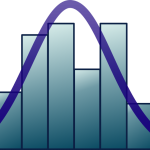# Quantitative Methods in Marine Science

University
Module
Teacher
Semester
Semester 1
Credits
6

Numerical tools help to ask scientific questions more efficiently and extract appropriate answers. This course will introduce students to a number of the basic techniques in data analysis and numerical modelling, to help them summarise a problem in mathematical terms, plan experiments or field sampling campaigns, and gather insights from the data collected.

Students will learn how to identify sources of variation in biological data and decide on sampling/experimental units and replicates. Major inferential statistical and data exploration techniques will be taught. Numerical models will be introduced as a way to simplify and formalise a system. The programming language (R) will be used to apply all those techniques. Students will learn:

•   how to use computer code to read and manipulate data, to implement statistical tests or dynamical models
•   how to efficiently plan an experiment or field sampling campaign
•   how to choose an appropriate data analysis technique
•   how to interpret the output of basic inferential statistics
•   how to represent data and model output graphically.

#### Course Contents

The class will consist of theoretical parts and applications to actual data sets. The themes tackled are presented below:

While the core of the programme will be the same in all universities, some classes are optional (in brackets: [] ) and the specific time spent on each part will vary between universities.

• Maths and programming basics notion of variable and of assignation; data types; data import; data manipulation, repetition of operations. numerical integration of differential equations; matrix computation data representation (plotting)
• Experimental/sampling design best practices in experiment and sampling design for optimal statistical power
• Linear model
• revision of simple linear regression, revision of ANOVA (as a particular case of linear model), multiple regression and multi-factor ANOVA; model selection
• introduction to generalised linear model: logistic regression, Poisson regression [introduction to mixed effects models]
• Non parametric tests notion of rank, basic non-parametric version of inferential tests (Wilcoxon-Mann-Whitney, Kruskall-Wallis) [notion of bootstrap and bootstrap tests]
• Introduction to multivariate data analysis
• Principal Component Analysis [Correspondence Analysis or Multidimensional Scaling]
• Numerical modelling 0D dynamical box and flux models (Fasham-like NPZD model)
• Population dynamics models (Leslie-like matrix models).

#### Final Competencies

How to translate a marine sciences question or hypothesis in mathematical terms and how to select the factors that are more relevant to answer it.

Further course information can be found here: https://studiekiezer.ugent.be/studiefiche/en/C003872/2022